﻿ MCMC算法及其应用

MCMC算法及其应用MCMC Algorithm and Its Application

Abstract: This paper mainly introduces the Markov Chain Monte Carlo Method (MCMC), which is mainly the Metropolis method, Hasting method and Gibbs method. The basic steps of these algorithms are in-troduced. At the same time, the convergence of Markov chain is used, the error of the algorithm is discussed, and the algorithm is improved. Finally, some simple applications of the limit properties are given. This paper is divided into six parts: The first part introduces the nature of the Markov chain. The second part introduces the Metropolis-Hasting (M-H) algorithm and promotion, and in-troduces two special MCMC algorithms, namely Metropolis algorithm and Hasting algorithm and algorithm implementation process. The third part introduces the improvement of the proposed probability distribution, which reduces the error and improves the convergence. The fourth part introduces the Gibbs algorithm and the Bayesian model. The fifth part gives examples and appli-cations, and finds a suitable implementation method among many methods. The last part, the sixth part, is summarized.

1. 前言

1.1. 马尔可夫链的基本概念

1.1.1. 马尔可夫链的定义

$P\left({X}_{t+1}={x}_{t+1}|{X}_{t}={x}_{t},\cdots ,{X}_{1}={x}_{1},{X}_{0}={x}_{0}\right)=P\left({X}_{t+1}={x}_{t+1}|{X}_{t}={x}_{t}\right)$

$p\left(x,y\right)$ 为过程从状态x转移到状态y的概率，称为一步转移概率； ${p}^{n}\left(x,y\right)$ 为过程从状态x经过n步转移后到达状态y的概率，称为n步转移概率。${\tau }_{y}=\text{inf}\left\{n\ge 1:{X}_{n}=y\right\}$，则 ${\tau }_{y}$ 是一个停时，称之为状态y的首中时，记

${f}^{n}\left(x,y\right)\triangleq {P}^{x}\left({\tau }_{y}=n\right),n\ge 1$

$f\left(x,y\right)\triangleq \underset{n\ge 1}{\sum }{f}^{n}\left(x,y\right)={P}^{x}\left({\tau }_{y}<\infty \right)$

1.1.2. 马尔可夫链的性质

1) 常返性。如果从状态x出发以概率1回到状态x，即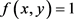，称状态x是常返的，否则称状态x时暂留的。如果一个马尔可夫链所有状态都是常返的，则称这个马尔可夫链是常返的。记 ${\mu }_{y}={E}^{y}\left[{\tau }_{y}\right]$

y的平均返回时间，如果平均回返时间 ${\mu }_{y}<\infty$，其中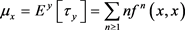2) 不可约性。如果所有状态都存在n，使得n步转移概率 ${p}^{n}\left(x,y\right)>0$，等价于每一个状态都可以经过有限步到达其他状态，也就是每个状态都是联通的，称这样的状态为不可约状态。有限状态的马尔可夫链是不可约的，则这个马尔可夫链是正回返的。

3) 周期性。n步转移概率 ${p}^{n}\left(x,y\right)$，状态x的周期数为 ${d}_{x}=\text{GCD}\left\{n\ge 1:pn\left(x,x\right)>0\right\}$

4) 遍历性。如果状态i是正常返、不可约、非周期的，则称该状态i是遍历的。所有状态都是遍历的马尔可夫链，称为遍历的马尔可夫链。

5) 平稳分布。E上一个概率分布 $\left(\pi \left(y\right):y\in E\right)$，如果满足 $\Pi P=\Pi$，即 ${\sum }_{x\in E}\pi \left(x\right)p\left(x,y\right)=\pi \left(y\right),\forall y\in E$，则称 $\Pi$ 为转移矩阵Ρ的平稳分布，显然有，也就是π是可逆的。

1.2. 马尔可夫链蒙特卡罗方法原理

2. Metropolis-Hasting(M-H)算法及推广

Metropolis算法是MCMC的核心，其实也就是改进的接受-拒绝抽样方法。接受-拒绝算法的具体步骤如下：

1) 首先选择一个容易抽样的分布作为建议概率分布，记为 $g\left(x\right)$，接着确定一个常数C，其中 $C>1$，使得在x的定义域上都有 $f\left(x\right)\le Cg\left(x\right)$

2) 生成服从建议概率密度函数的 $g\left(x\right)$ 的随机数y。

3) 接着生成一个服从均匀分布 $U\left(0,1\right)$ 的随机数u。

4) 计算接受概率 $h\left(x\right)=\frac{f\left(x\right)}{Cg\left(x\right)}$，如果 $u，则接受y，否则继续转到第(2)步。

5) 不断重复(2)~(4)就可以生成一组服从目标分布 f ( x) 的随机数序列。

1) 首先构造建议概率分布 $g\left(\text{ }\cdot \text{ }|{x}_{t}\right)$，并从建议概率分布 $g\left(\text{ }\cdot \text{ }|{x}_{t}\right)$，其中 $g\left(y|x\right)=g\left(x|y\right)$，产生一个随机数y作为下一个状态。

2) 接着按从均匀分布 $U\left(0,1\right)$ 的随机数u，如果，则接受该建议的随机数 ，即 ${x}_{t+1}=y$，否则 ${x}_{t+1}={x}_{t}$

3) 重复上述过程，我们就可以得到一组仅依赖于上一个随机数并且与前面随机数无关的随机序列。

4) 计算接受概率 $\alpha \left({x}_{t},y\right)=\frac{f\left(y\right)}{f\left({x}_{t}\right)}$

Metropolis算法的应用受到了建议概率分布的对称形式的限制，因此，Hasting把建议概率分布从对称分布形式推广到一般情况，形成了Hasting算法，也称之为通用梅特罗波利斯算法，此时接受概率变为

$\alpha \left({x}_{t},y\right)=\mathrm{min}\left(1,\frac{f\left(x\right)g\left({x}_{t}|y\right)}{f\left(y\right)g\left(y|{x}_{t}\right)}\right)$

2.1. 收敛性证明

1) $q\left(y|x\right)$ 不可约和非周期，则 $A\left(x,y\right)=q\left(y|x\right)\alpha \left(y,x\right)$ 也不可约且非周期的。

2) $A\left(x,y\right)$ 可逆且满足细致平衡条件。

3)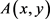满足不变性。

Proof. 1) 由马尔可夫链性质知，当马尔可夫链满足不可约性和非周期性，那么m步转移概率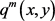产生的任何m步跟踪路径为大于零的一个值，由于m步转移函数 ${A}^{m}\left(x,y\right)={\left(q\left(y|x\right)\alpha \left(y,x\right)\right)}^{m}={\left(q\left(y|x\right)\right)}^{m}{\left(\alpha \left(y,x\right)\right)}^{m}$ 具有正概率，所以 $A\left(x,y\right)=q\left(y|x\right)\alpha \left(x,y\right)$ 也不可约且非周期的。

2) 因为

$\begin{array}{c}f\left(x\right)A\left(x,y\right)=f\left(x\right)q\left(y|x\right)\alpha \left(y,x\right)\\ =f\left(x\right)q\left(y|x\right)\text{min}\left\{1,f\left(y\right)/f\left(x\right)\right\}\\ =\text{min}\left\{f\left(x\right)q\left(y|x\right),f\left(y\right)q\left(y|x\right)\right\}\\ =f\left(y\right)q\left(y|x\right)\text{min}\left\{f\left(x\right)/f\left(y\right),1\right\}\\ =f\left(y\right)q\left(y|x\right)\alpha \left(x,y\right)=f\left(y\right)A\left(y,x\right)\end{array}$

3) 根据马尔可夫链的性质知，满足细致平衡性也就确保了不变性，所以就可以达到整个马尔可夫链总体的平衡  。下面要证明马尔可夫链满足不变性也就是满足

$f\left(x\right)A\left(x,y\right)=f\left(x\right)$

$\underset{x}{\sum }f\left(x\right)A\left(x,y\right)=\underset{x}{\sum }f\left(y\right)A\left(y,x\right)=f\left(y\right)\underset{x}{\sum }A\left(y,x\right)=f\left(y\right)$

$\int f\left(x\right)A\left(x,y\right)\text{d}x=\int f\left(y\right)A\left(y,x\right)\text{d}x=f\left(y\right)\int A\left(y,x\right)\text{d}x=f\left(y\right),$

2.2. 例子

3. 建议概率分布改进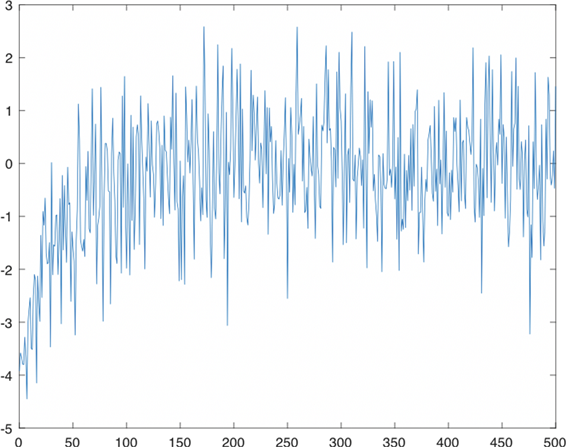Figure 1. Acceptance probability varies with sample size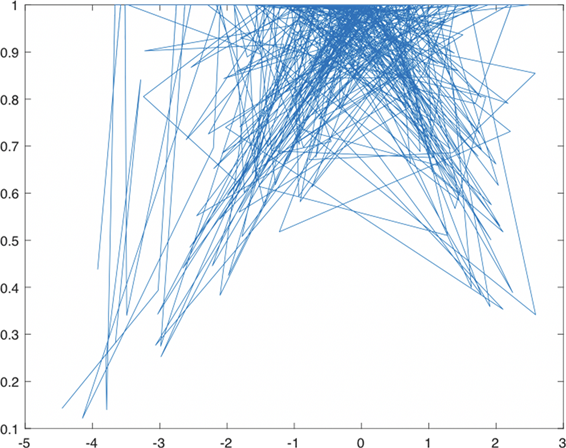Figure 2. Acceptance probability varies with x

1) 首先从建议概率分布 $q\left(y|{x}_{t}\right)$ 抽样产生样本值 ${Z}_{t}$，记候选样本值 ${Y}_{t}={X}_{t}+{Z}_{t}$

2) 接着按从均匀分布 $U\left(0,1\right)$ 的随机数u，如果 $u\le \alpha \left({X}_{t},{Y}_{t}\right)$，则接受该建议的随机数Yt，即 ${X}_{t+1}={Y}_{t}$，否则 ${X}_{t+1}={X}_{t}$

3) 重复上述过程，得到一组仅依赖于上一个随机数并且与前面随机数无关的

4) 计算接受概率 $\alpha \left(X,Y\right)=\mathrm{min}\left(1,\frac{f\left({Y}_{t}\right)q\left({X}_{t}|{Y}_{t}\right)}{f\left({X}_{t}\right)q\left({Y}_{t}|{X}_{t}\right)}\right)=\mathrm{min}\left(1,\frac{f\left({Y}_{t}\right)}{f\left({X}_{t}\right)}\right)$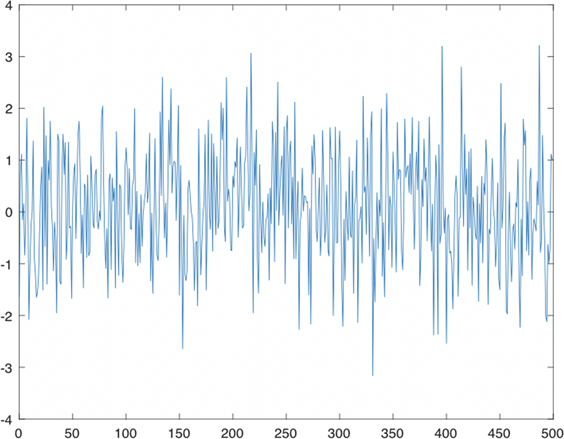Figure 3. Acceptance probability varies with sample size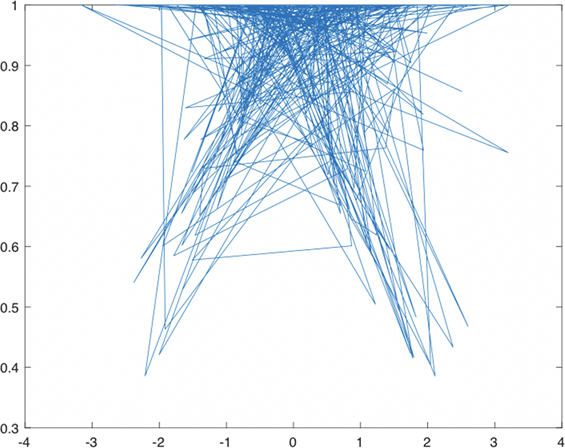Figure 4. Acceptance probability varies with x

4. Gibbs方法

Gibbs方法是将高维问题  化为一维问题，在对每个分量进行抽样的时候等价于对其他所有分量的条件分布进行抽样。所以下面将随机变量X分解成单随机变量 $X=\left({X}_{1},{X}_{2},\cdots ,{X}_{m}\right)$，随机变量X的概率分布 $f\left(x\right)=f\left({x}_{1},{x}_{2},\cdots ,{x}_{m}\right)$，单个随机变量 ${x}_{j}$ 的全条件概率分布

$f\left({x}_{j}|{x}_{-j}\right)=f\left({x}_{j}|{x}_{1},{x}_{2},\cdots ,{x}_{j-1},{x}_{j+1},\cdots ,{x}_{m}\right),j=1,2,\cdots ,m$

Gibbs算法就是

1) 将已知的概率分布分解为多个单变量的全条件概率分布。

2) 通过对多个单变量全条件概率分布中产生候选点，从 $f\left({x}_{j}|{x}_{-j}\right)$，其中， $j=1,2,\cdots ,m$ 中产生候选点 ${X}_{j}\left(t\right)$，更新 ${x}_{j}={X}_{j}\left(t\right)$，来实现对已知概率分布的抽样。

(其中 $i>j$，即 $\mu <\lambda$ )，求在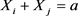的情况下 ${X}_{i}$ 的条件分布 ${f}_{{X}_{i}|{X}_{i}+{X}_{j}}\left(x|a\right)=c\mathrm{exp}\left\{-\left(\lambda -\mu \right)x\right\}$$0。令初始状态 $\left({x}_{1},{x}_{2},\cdots ,{x}_{5}\right)$ 是和等于15的任意五个整数。从1，2，3，4，5中随机取出两个整数，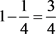的指数分布值，下面令等于这个值，再重新设 ${x}_{5}$ 的值使得 ${\sum }_{i=1}^{5}{X}_{i}=15$。重复上述过程就可以得

5. 实例与应用

$\left(\Omega ,F,P,\left\{{\mathcal{F}}_{t}\right\}\right)$ 是一个带 $\sigma$ 域流的概率空间  ，P为风险中性测度且服从 $\text{d}{S}_{t}=r{S}_{t}\text{d}t+\sigma {S}_{t}\text{d}{X}_{t}$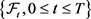为P测度下产生的自然 $\sigma$ 域流。设St为股票价格， ${S}_{0}=S$，无红利美式股票卖权协议价格为K，期限为T，无风险利率r， $\sigma$ 为股票波动率， ${Z}_{t}$ 为标准布朗运动。令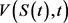表示股票在时刻t的价格，所以我们可以构造一种投资组合来得到 $V\left(S\left(t\right),t\right)$，利用 $\Delta$ 对冲技巧，可以给出期权定价的数学模型，形成投资组合 $\Pi =V-\Delta S$，选取适当的 $\Delta$ 使得在 $\left(t,t+\text{d}t\right)$ 时段内， $\Pi$ 无风险。设在时刻t形成的投资组合

$\Pi$，并在 $\left(t,t+\text{d}t\right)$ 内，不改变份额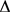，由于 $\Pi$ 无风险，在时刻 $t+\text{d}t$，投资组合的回报 $\frac{{\Pi }_{t+\text{d}t}-{\Pi }_{t}}{{\Pi }_{t}}=r\text{d}t$

$\text{d}t{V}_{t}-\Delta \text{d}{S}_{t}={\Pi }_{t+\text{d}t}-{\Pi }_{t}=r{\Pi }_{t}\text{d}t=r\left({V}_{t}-\Delta {S}_{t}\right)\text{d}t$。运用Itǒ公式，并且代入上式，得到Black-Scholes方程：

$\frac{\partial V}{\partial t}+\frac{1}{2}{\sigma }^{2}{S}^{2}\frac{{\partial }^{2}V}{\partial {S}^{2}}+rs\frac{\partial V}{\partial S}-rV=0$

$V\left(s\left(t\right),t\right)=\text{exp}\left\{-r\left(T-t\right)\right\}{\int }_{-\infty }^{\infty }{\left(x-K\right)}^{+}f\left(s,s\left(t\right)\right)\text{d}s$ 其中， $f\left(s,s\left(t\right)\right)$ 表示给定当前资产为 $s\left(t\right)$，资产价格S在期权到期日T时的密度函数。因此股票价格波动是一个随机过程，股票价格为 ${S}_{t}={S}_{t-1}\mathrm{exp}\left\{\left(r-{\sigma }^{2}/2\right)\Delta t+\sigma \sqrt{\Delta t}{X}_{t}\right\}$。其中， $\left\{{X}_{t}\right\}$ 为相互独立的服从 {Xt} 的随机变量。c表示该期权在零时刻的价格，因此 $c=\text{exp}\left\{-rT\right\}E{\left[{S}_{0}-K\right]}^{+}$，由于这个期权是一种路径依赖的期权，计算包含一个多重积分，积分的维数为n，所以求解过程很复杂，因此采用马尔可夫链蒙特卡罗方法求解。

$f\left(x\right)\propto H\left(x\right)\text{exp}\left\{-{x}^{\text{T}}{}^{x}x/2\right\}$ ,

$d={\left(\frac{{Z}_{1}}{‖Z‖},\frac{{Z}_{2}}{‖Z‖},\cdots ,\frac{{Z}_{s}}{‖Z‖}\right)}^{\text{T}}$

$‖Z‖=\sqrt{{Z}_{1}^{2}+{Z}_{2}^{2}+\cdots +{Z}_{s}^{2}},\text{\hspace{0.17em}}{Z}_{1},{Z}_{2},\cdots ,{Z}_{s}\sim N\left(0,1\right)$

1) s维单位超球体上均匀分布抽样产生随机方向d。

2) $q\left(\lambda |d,{X}_{t}\right)$ 抽样产生 $\lambda$，计算 ${Y}_{t}={X}_{t}+\lambda d$

3) 若 $U\le \alpha \left({X}_{t},{Y}_{t}\right)$${X}_{t+1}={Y}_{t}$；否则， ${X}_{t+1}={X}_{t}$

1) 取记号a1，a2，其中 $a1=\mathrm{exp}\left\{\left(r-0.5\ast {\sigma }^{2}\right)\ast h\right\}$$a2=\sigma \ast \sqrt{X}$，计算a1，a2；

2) 若 $S\left(T\right)>K$，则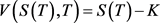，否则 V (S (T ),T ) = 0 ；

3) 计算价格 $S\left(t\right)$。这里取利率 $r=0.05$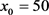$\sigma =0.3$$T=1$$n=16$$npath=25000$$K=55$，可以求解到股票价格 $S=25.2245$

6. 总结

A 附录1

function [x,m] = rw(x0,a,iter)

x=x0;

for t=0:(iter+1)

y=rand () *2*a-a+x;

m=min(1, exp((x^2-y^2)/2));

u=rand ();

if u<=m

x=y;

end

end

x0=-4;

>> a=0.5;

>> n=500;

>> x=zeros(1, n+1);

>> iter= [0:1: n];

>> for t=0: n

plot(iter,x)

plot(x,m)

B 附录2

function [x,m] = rws(x0,a,iter)

x=x0;

for t=0:(iter+1)

u1=rand ();

u2=rand ();

u=rand ();

x=(-2*log(u1))^(1/2)*cos(2*pi*u2);

y=x+a*(2*u-1);

m=min(1, exp((-x^2+y^2)/2));

if u<=m

x=y;

end

end

x0=-4;

>> a=0.5;

>> n=500;

>> x=zeros(1, n+1);

>> iter=[0:1: n];

>> for t=0: n

plot(iter,x)

plot(x,m)

C 附录3

function [price1, price2, x]=MCMC(r,x,sigma,T,n,npath,K)

h=T/n;

xc=0;

a1=exp((r-0.5*sigma^2) *h);

a2=sigma*sqrt(h);

s1=0;

s2=0;

for i=1: npath

z=normrnd (0,1);

x=x*a1*exp(a2*z);

xc=xc+x;

i=i+1;

end

if x>K

R=x-K;

else

R=0;

end

s1=s1+R;

s2=s2+R^2;

theta=s1/npath;

stderr=sqrt((s2-s1^2/npath)/npath/(npath-1));

if x-K>0

price1=exp(-r*T) *theta;

price2=0;

else

price2=exp(-r*T) *stderr;

price1=0;

end

price1 = 25.2245

price2 = 0

x= 6.6300e+05

 Hasting, W. (1970) Monte Carlo Sampling Methods Using Markov Chains and Their Applications. Biometrika, 57, 97-109.
https://doi.org/10.1093/biomet/57.1.97

 刘军. 科学计算中的蒙特卡罗策略[M]. 北京: 高等教育出版社, 2005.

 Rubinstein, R. (1981) Simulation and Monte Carlo Method. John Wiley, New York.

 Chib, S. and Greenberg, E. (1995) Understanding the Metropolis-Hasting Algorithm. Taylor and Francis, 49, 327-335.

 Tierney, L. (1994) Markov Chains for Exploring Posterior Distributions. The Annals of Statistics, 22, 1701-1728.
https://doi.org/10.1214/aos/1176325750

 Andrieu, C. (2003) An Introduction to MCMC for Machine Learning. Machine Learning, 50, 5-43.
https://doi.org/10.1023/A:1020281327116

 Brooks, S., Gelman, A. and Jones, L. (2011) Handbook of Markov Chain Monte Carlo. A Chapman and Hall Book.

 Roberts, G. (2004) General State Space Markov Chains and MCMC Algorithms. Probability Surveys, 1, 20-71.
https://doi.org/10.1214/154957804100000024

 Liang, F. and Liu, C. (2010) Advanced Markov Chain Monte Carlo Methods. John and Sons Ltd. Publication.
https://doi.org/10.1002/9780470669723

 康崇禄. 蒙特卡罗方法理论和应用[M]. 北京: 科学出版社, 2014.

 肖柳青. 随机模拟方法与应用[M]. 北京: 北京大学出版社, 2014.

 张波. 应用随机过程[M]. 北京: 中国人民大学出版社, 2017.

 Dagpunar, J. (2007) Simulation and Monte Carlo: With Applications in Finance and MCMC. John Wiley and Sons Ltd.

 Lyas, A. (2016) Importance Sampling for Least-Square Monte Carlo Methods. Degree Project in Mathematics.

Top